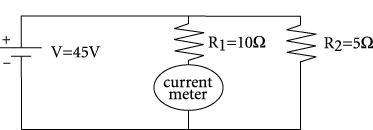The current through the meter is equal to the current through $$R_1$$. The current through $$R_1$$ is $$\frac{\mathrm{45V} }{\mathrm{10 \Omega } } = 4.5A$$